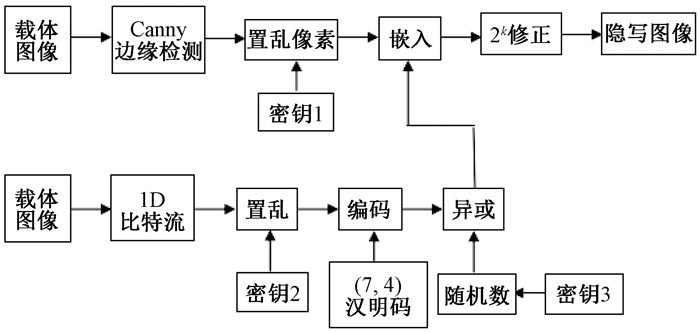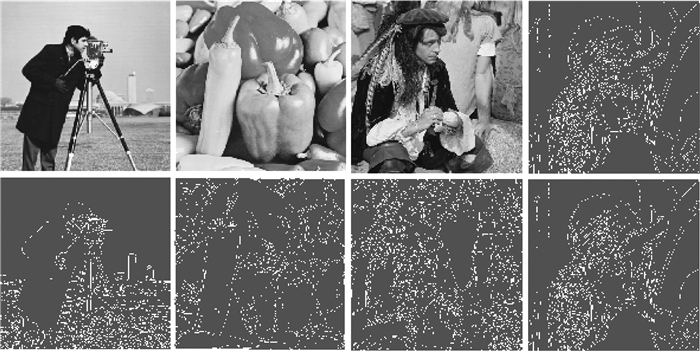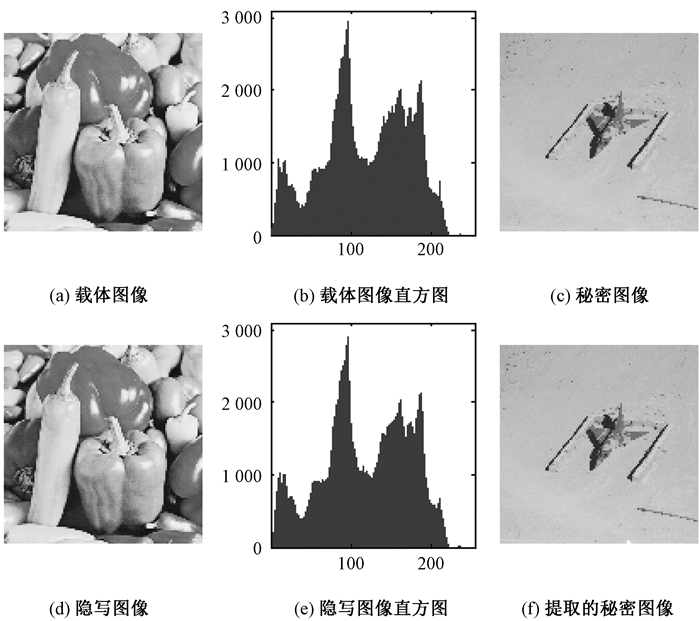﻿ 基于汉明码和Logistic-正弦映射的图像隐写
 郑州大学学报（理学版）2018, Vol. 50Issue (3): 22-26  DOI: 10.13705/j.issn.1671-6841.2017254### 引用本文LIU Yinghong, SUN Shuliang. Image Steganography Based on Hamming Code and Logistic-sine Encryption[J]. Journal of Zhengzhou University(Natural Science Edition), 2018, 50(3): 22-26.### 文章历史

Image Steganography Based on Hamming Code and Logistic-sine Encryption
LIU Yinghong, SUN ShuliangSchool of Electronic and Information Engineering, Fuqing Branch of Fujian Normal University, Fuqing 350300, China
Abstract: A novel steganographic algorithm based on Hamming code and Logistic-sine map was proposed. Canny edge detector was applied to detect the edge of cover image. Then three keys were produced with Logistic-sine map. Then Hamming encoding was executed to code the secret data and embedded in scrambled edge pixels. Finally, the method of 2k correction was used to achieve better imperceptibility in stego image. Experimental results showed that the proposed algorithm was excellent in security, availability and robustness.
Key words: Hamming code    2k correction    Logistic-sine map    Canny detector
0 引言

1 Logistic-正弦映射 1.1 Logistic映射

Logistic映射是最简单常用的一维混沌映射.表达式为xn+1=L(μ, xn)=μxn(1－xn)，参数μ的取值范围是[3.57, 4].但在这个区间内，也并不是所有值都能使系统处于混沌状态.Logistic映射的李雅普诺夫指数较小，而且生成的混沌序列分布并不均匀，这些都是Logistic映射存在的不足之处.

1.2 正弦映射
 ${x_{n + 1}} = S(\mu, {x_n}) = \mu \;{\rm{sin}}(\pi {x_n})/4,$ (1)

1.3 Logistic-正弦映射
 ${x_{n + 1}} = (L(\mu, {x_n}) + S(\left( {4-\mu } \right), {x_n})){\rm{mod}}\;1 \\= (\mu {x_n}(1-{x_n}) + \left( {4-\mu } \right){\rm{sin}}({\rm{ \mathsf{ π} }}{x_n})/4){\rm{mod}}\;1,$ (2)

2 汉明码

 ${2^r}-1 \ge n\;或者\;{2^r}-1 \ge k + r,$ (3)表 1 校验位与数据位之间的关系 Table 1 Relationship between parity bit and data bit

 ${p_1} = {m_1} \oplus {m_2} \oplus {m_4}, {p_2} = {m_1} \oplus {m_3} \oplus {m_4}, {p_3} = {m_2} \oplus {m_3} \oplus {m_4},$

“⊕”表示异或操作.表 2 (7, 4)汉明码的码字 Table 2 Codewords of (7, 4) Hamming code

 $\mathit{\boldsymbol{R}} = \left( {\mathit{\boldsymbol{M}} \times \mathit{\boldsymbol{G}}} \right){\rm{mod}}\;2,$ (4)

 $\mathit{\boldsymbol{G}}{\rm{ = }}\left[{\begin{array}{*{20}{c}} 1&1&1&0&0&0&0\\ 1&0&0&1&1&0&0\\ 0&1&0&1&0&1&0\\ 1&1&0&1&0&0&1 \end{array}} \right].$

 $\mathit{\boldsymbol{Z}} = (\mathit{\boldsymbol{R}} \times {\mathit{\boldsymbol{H}}^{\rm{T}}})\;{\rm{mod}}\;2,$ (5)

 $\mathit{\boldsymbol{H}}{\rm{ = }}\left[{\begin{array}{*{20}{c}} 1&0&1&0&1&0&1\\ 0&1&1&0&0&1&1\\ 0&0&0&1&1&1&1 \end{array}} \right];$

3 秘密信息隐藏算法图 1 信息隐藏过程框图 Figure 1 Diagram of data hiding process

1) 读取载体图像和密码图像.

2) 用Canny边缘检测算子检测载体图像边缘.

3) 用Logistic-正弦映射产生密钥1、密钥2和密钥3.

4) 用密钥1置乱边界像素.

5) 秘密图像转换为1D比特流.

6) 用密钥2置乱1D比特流.

7) 用(7, 4)汉明码编码1D比特流，并与用密钥3产生的随机数进行异或操作.

8) 比特流嵌入置乱后的边缘像素.

9) 用2k修正算法提高图像的视觉效果.

10)最终获得隐写图像.

4 实验过程和结果

 $MSE = \frac{1}{{MN}}\sum\limits_{i = 0}^{M-1} {\sum\limits_{j = 0}^{N-1} {{{\left\| {\mathit{\boldsymbol{I}}\left( {i, j} \right)-\mathit{\boldsymbol{S}}\left( {i, j} \right)} \right\|}^2}} },$

 $PSNR = 20\;{\rm{lo}}{{\rm{g}}_{10}}\left( {\frac{{255}}{{\sqrt {MSE} }}} \right).$ (6)

 $\bar x = \frac{1}{N}\sum\limits_{i = 1}^N {{x_i}} ;{\rm{ }} = \frac{1}{N}\sum\limits_{i = 1}^N {{y_i}} ;{\rm{ }}x = \{ {x_i}\left| {i = 1,{\rm{ }}2, \ldots ,{\rm{ }}N} \right.\} ;{\rm{ }}y = \{ {y_i}\left| {i = 1,{\rm{ }}2, \ldots ,{\rm{ }}N} \right.\} ;$
 ${\sigma _x}^2 = \frac{1}{{N - 1}}\sum\limits_{i = 1}^N {{{({x_i} - \bar x)}^2}} ;{\sigma _y}^2 = \frac{1}{{N - 1}}\sum\limits_{i = 1}^N {{{({y_i} - \bar y)}^2}} ;\\{\sigma _{xy}} = \frac{1}{{N - 1}}\sum\limits_{i = 1}^N {({x_i} - \bar x)({y_i} - \bar y)} .$图 2 Canny算子检测的载体边缘 Figure 2 Edge images with Canny detector表 3 三种参数的比较 Table 3 Comparison of three parameters图 3 隐写图像和提取的秘密图像 Figure 3 Stego image and retrieved image
5 结论

  韩涛, 祝跃飞. 基于Canny边缘检测的自适应空域隐写术[J]. 电子与信息学报, 2015, 37(5): 1266-1270. DOI:10.11999/JEIT141121 (0)  SUN S L. A novel edge based image steganography with 2k correction and Huffman encoding[J]. Information processing letters, 2016, 116(2): 93-99. DOI:10.1016/j.ipl.2015.09.016 (0)  ZHANG Y, JIANG J, ZHA Y, et al. Research on embedding capacity and efficiency of information hiding based on digital images[J]. International journal of intelligence science, 2013, 3(2): 77-85. DOI:10.4236/ijis.2013.32009 (0)  BAI J, CHANG C C. A high payload steganographic scheme for compressed images with hamming code[J]. International journal of network security, 2016, 18(6): 1122-1129. (0)  KIM C, SHIN D, SHIN D. Intelligent Information and Database Systems[M]. Heidelberg: Springer, 2011. (0)  ZHOU Y, BAO L, CHEN C L P. A new 1D chaotic system for image encryption[J]. Signal processing, 2014, 97(7): 172-182. (0)  HAMMING R W. Error detecting and error correcting codes[J]. Bell system technical journal, 2014, 29(2): 147-160. (0)  朱雪秀, 刘九芬, 张卫明. 一种基于汉明码和湿纸码的隐写算法[J]. 电子与信息学报, 2010, 32(1): 162-165. (0)  WANG Z, BOVIK A C. A universal image quality index[J]. IEEE signal processing letters, 2002, 9(3): 81-84. DOI:10.1109/97.995823 (0)  YU J G, YOON E J, SHIN S H, et al. A new image steganography based on 2k correction and edge-detection [C]// Information Technology: New Generations. New York, 2008: 563-568. (0)